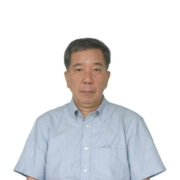7-1 Differential polynomials – Ex. 1Introduction to Ordinary Differential Equations
201 个评分Korea Advanced Institute of Science and Technology(KAIST)

Introduction to Ordinary Differential Equations

201 个评分

Linear Second order equations 3

与讲师见面

•Kwon, Kil Hyun
Professor
Department of Mathematical Sciences